# 82. The Vegetarian Resource Group commissioned Harris Poll in 2016 to conduct a nationally repre-sentative online poll of 2,015 adults aged 18 and over. They found that 3.3% of the respondents(a) Construct and interpret a 95% confidence interval for the proportion of vegetarians.(b) Explain why a 90% confidence interval for the proportion of vegetarians will be wider/narrower(c) Explain why the interval will be wider/narrower than the one in (a) if the sample sizeidentified as vegetarian.than the one in (a). Construct the interval to confirm.changed to n =300. Construct the interval to confirm.83. Choose the correct word(s) to fill in the blank for each sentence.(a) The hypotheses are always stated in terms of(b) Any numbers in the hypotheses come from the(olan (c) The test statistic and p-value assume the(statistics/parameters)(data/research question)(null/alternative/data) is true(null/alternative) hypothesis(d) The conclusion is always in terms of the84. Choose the correct word(s) to fill in the blank for each sentence.akgosq (a) If the null is true in reality, we would expect the test statistic tO De(small/large)(b) If the alternative is true in reality, we would expect the test statistic to be(small/large)nothing else changed besides an increase in sample size, the test statistic would be(smaller/larger)(d) If nothing else changed besides an increase in sample size, the p-value in a two sided testwould be(smaller/larger)05. According to NAFSA, 1.55% of all college students in the US studied abroad during the 2014-2015academic year. Being the location of our country's capitol, a researcher believes that a nigieiproportion of students study abroad in the District of Columbia than elsewhere in the country.Suppose a random sample of 250 college students from D.C. had 15 who studied abroad.(a) State the hypotheses in symbols and words to test the researcher's claim.(b) Calculate the test statistic.(c) Calculate the p-value.(d) Interpret the p-value.(e) State the conclusion of the test.86. Movie theaters make as much as 85% profit on items sold at the concession stand. Withoutsnacks at the movies, there would be no movies! A theater owner suspected that less than half ofhis customers purchased food at the concession counter. To examine this claim, a random sampleof 80 patrons was selected and 35 of them purchased food.(a) State the hypotheses in symbols and words to test the theater owner's claim.(b) Calculate the test statistic.(c) Calculate the p-value.(d) Interpret the p-value.(e) State the conclusion of the test.

Question
1 views

Question 85 parts A, B, and C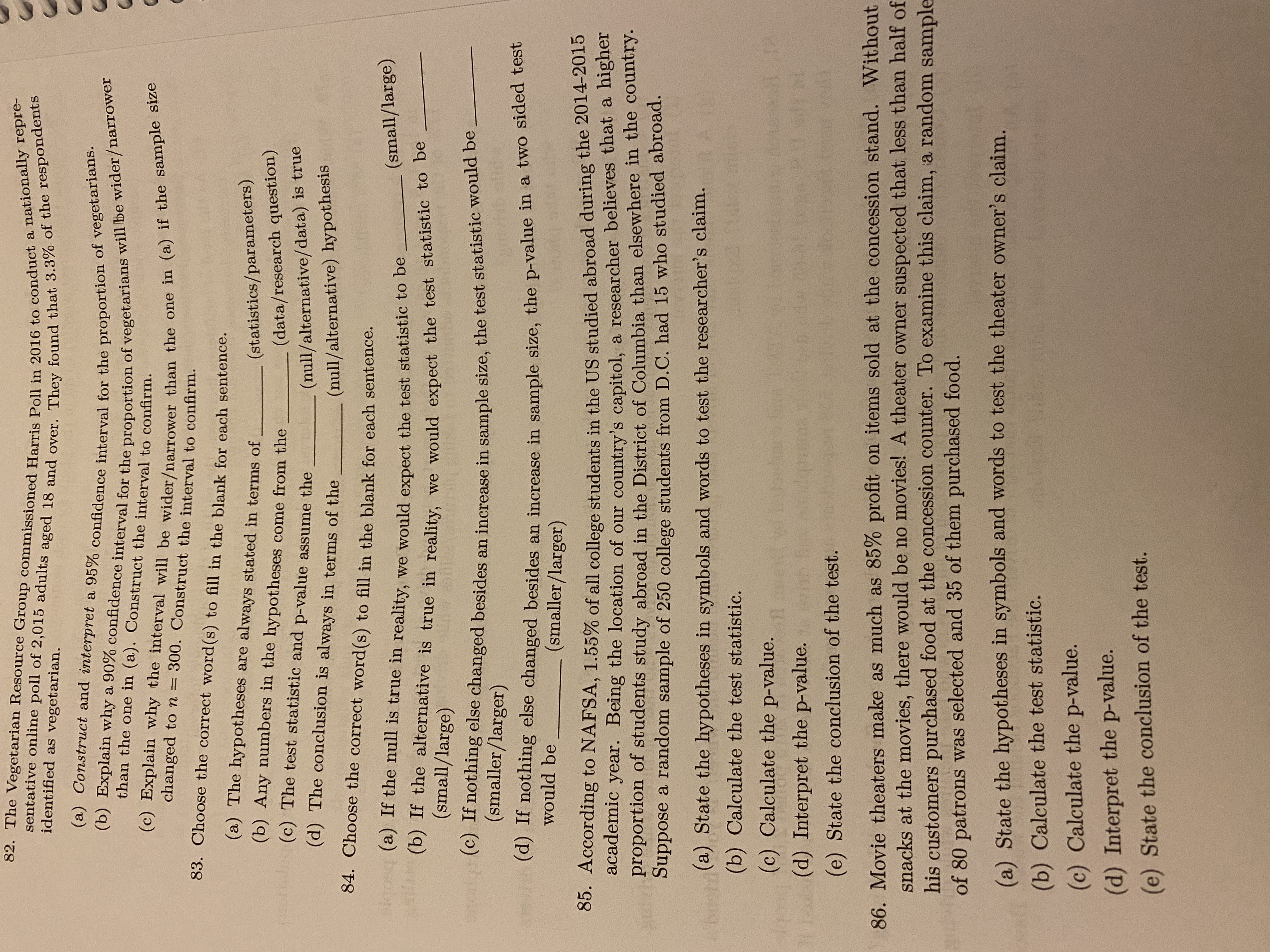help_outlineImage Transcriptionclose82. The Vegetarian Resource Group commissioned Harris Poll in 2016 to conduct a nationally repre- sentative online poll of 2,015 adults aged 18 and over. They found that 3.3% of the respondents (a) Construct and interpret a 95% confidence interval for the proportion of vegetarians. (b) Explain why a 90% confidence interval for the proportion of vegetarians will be wider/narrower (c) Explain why the interval will be wider/narrower than the one in (a) if the sample size identified as vegetarian. than the one in (a). Construct the interval to confirm. changed to n = 300. Construct the interval to confirm. 83. Choose the correct word(s) to fill in the blank for each sentence. (a) The hypotheses are always stated in terms of (b) Any numbers in the hypotheses come from the (olan (c) The test statistic and p-value assume the (statistics/parameters) (data/research question) (null/alternative/data) is true (null/alternative) hypothesis (d) The conclusion is always in terms of the 84. Choose the correct word(s) to fill in the blank for each sentence. akgosq (a) If the null is true in reality, we would expect the test statistic tO De (small/large) (b) If the alternative is true in reality, we would expect the test statistic to be (small/large) nothing else changed besides an increase in sample size, the test statistic would be (smaller/larger) (d) If nothing else changed besides an increase in sample size, the p-value in a two sided test would be (smaller/larger) 05. According to NAFSA, 1.55% of all college students in the US studied abroad during the 2014-2015 academic year. Being the location of our country's capitol, a researcher believes that a nigiei proportion of students study abroad in the District of Columbia than elsewhere in the country. Suppose a random sample of 250 college students from D.C. had 15 who studied abroad. (a) State the hypotheses in symbols and words to test the researcher's claim. (b) Calculate the test statistic. (c) Calculate the p-value. (d) Interpret the p-value. (e) State the conclusion of the test. 86. Movie theaters make as much as 85% profit on items sold at the concession stand. Without snacks at the movies, there would be no movies! A theater owner suspected that less than half of his customers purchased food at the concession counter. To examine this claim, a random sample of 80 patrons was selected and 35 of them purchased food. (a) State the hypotheses in symbols and words to test the theater owner's claim. (b) Calculate the test statistic. (c) Calculate the p-value. (d) Interpret the p-value. (e) State the conclusion of the test. fullscreen
check_circle

Step 1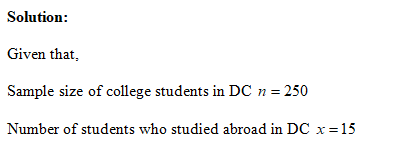Step 2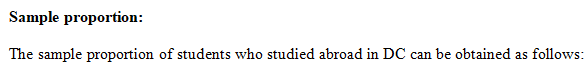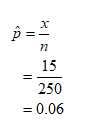Step 3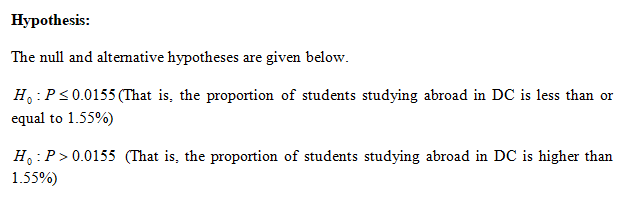...

### Want to see the full answer?

See Solution

#### Want to see this answer and more?

Solutions are written by subject experts who are available 24/7. Questions are typically answered within 1 hour.*

See Solution
*Response times may vary by subject and question.
Tagged in